•研究论文
• 由于毕设题目是与无人机相关，于是借此机会了解一些无人机平台自主协同的相关知识，这篇文章是读《无人机编队飞行技术》之后的一些简单的概念总结。 全篇书名《无人机编队飞行技术》-王新民、王晓燕、肖堃 基本...

由于毕设题目是与无人机相关，于是借此机会了解一些无人机平台自主协同的相关知识，这篇文章是读《无人机编队飞行技术》之后的一些简单的概念总结。
全篇书名《无人机编队飞行技术》-王新民、王晓燕、肖堃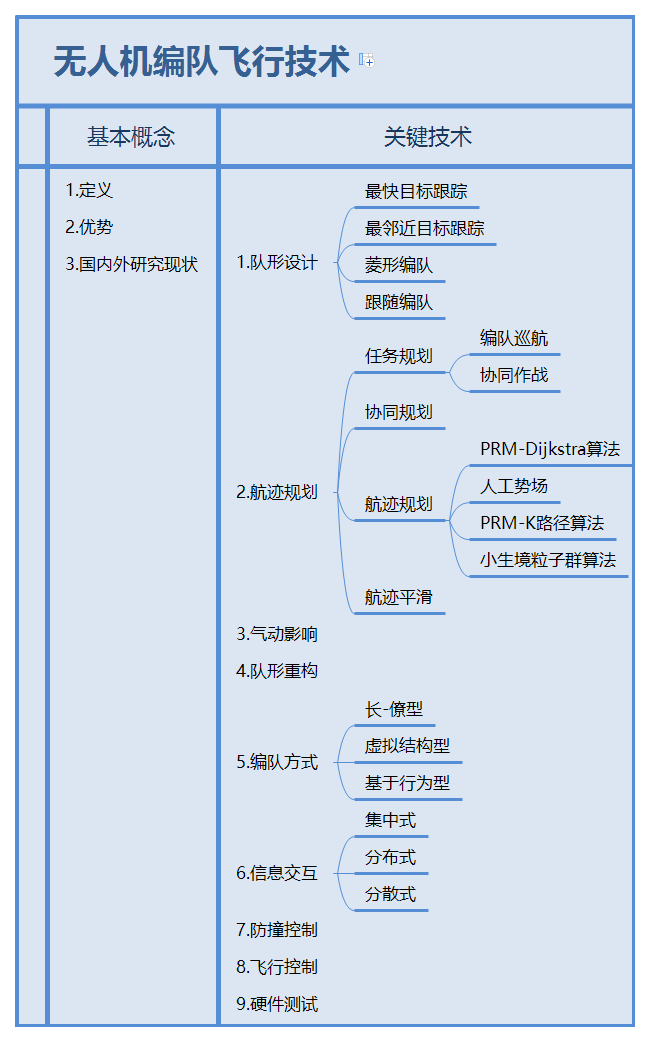基本概念
无人机编队飞行定义
无人机的编队飞行，是指两架或两架以上具有自主功能的无人机为适应任务要求而进行的一定结构的某种队形排列和任务分配的组织模式。它既包括编队飞行的队形设计、飞行过程中的队形保持和根据外部情况及任务需求进行队形的动态调整变化，也包括飞行任务的规划和组织，以体现整个无人机群的协同一致性。其中，带队的那架无人机称为长机，而其余的无人机称为僚机。
无人机编队飞行的优势

可以扩大视野
可以高精度定位，多角度成像
可用于演示验证星载设备
可提高无人机的整体效率
可以提高命中率
可以减小整体的飞行阻力

关键技术
最快目标跟踪与最邻近目标跟踪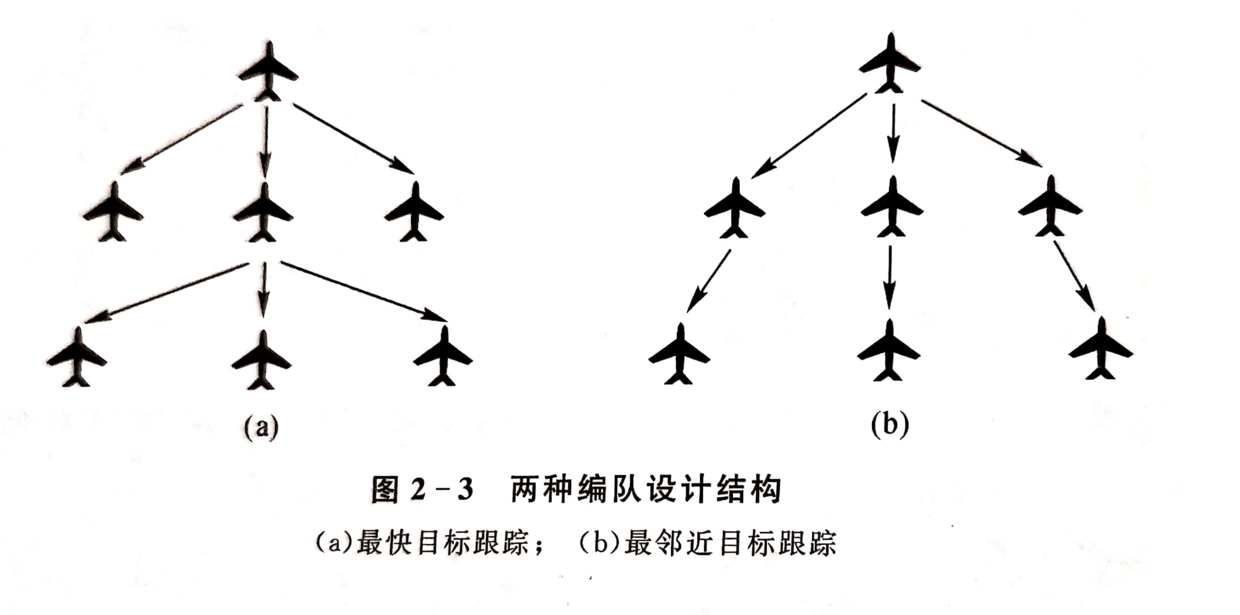队形重构的研究内容
怎样在飞行中实时调整无人机位置使得性能良好的无人机能在飞行中完成对编队队形的重构，从而保持队形不变或者达到最优队形。
航迹规划结构框图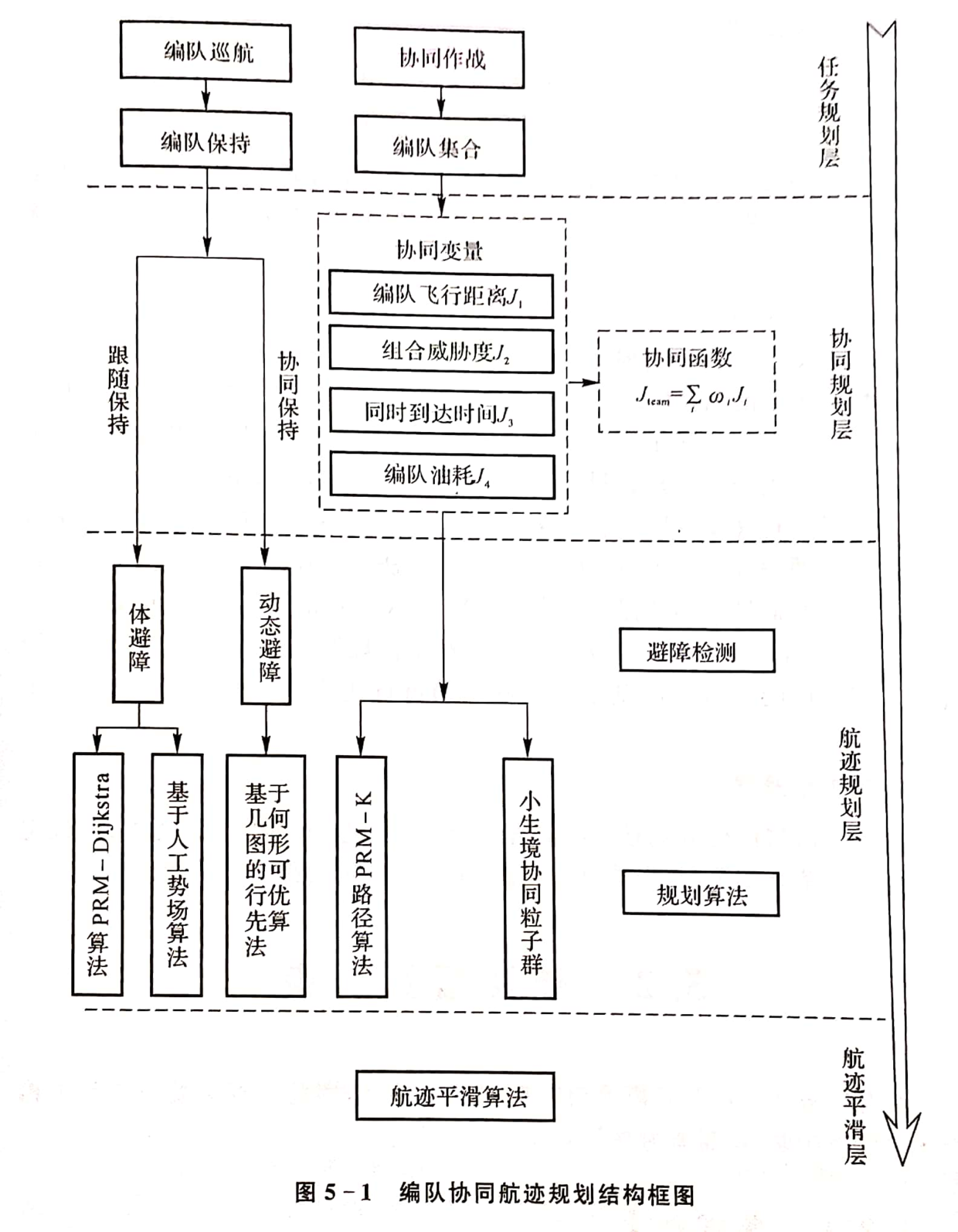航迹规划层的目的
根据任务要求、威胁分布、无人机机动特性、燃料限制，选择K条能使无人机回避地方威胁，安全完成预定任务的飞行路径，规划结果用一系列航路点表示。
航迹规划算法
概率地图算法——PRM
Dijkstra算法
Dijkstra算法作为一种最小航迹选择算法，能够在短时间内对节点遍历搜索，在可行解中寻求到最优解，使无人机的飞行航迹最短。
人工势场APF算法
人工势场的基本思想是将机器人在周围环境中的运动，设计成一种抽象的人造引力场中的运动，目标点对移动机器人产生的“引力”，障碍物对移动机器人产生“斥力”，最后通过求合力来控制移动机器人的运动。
K算法
K算法是在Dijkstra算法基础上，不只求出最短路径，还要求出次优化的K条路径。
小生境粒子群算法
基于小生境粒子群的无人机编队协同航迹规划方法，首先满足各种航迹约束的条件下，序贯产生粒子构成航迹集合，避免种群中粒子的有效性测试，无须进行多次初始化；通过引入改进的聚类小生境生成策略，在航迹规划空间内构造不同的相互独立的小生境种群；在每个小生境粒群体中利用粒子群算法进行速度和位置的更新，每个粒子群的全局极值尽在自己的小生境群体中起作用，追逐不同的极值点；并且引入种群淘汰策略，每隔一定代数，对陷入局部最优的最劣子种群进行随机初始化，从而避免了算法早熟收敛，保证了收敛到全局最优。当进化过程中航迹代价函数趋于稳定或达到最大迭代次数时，进行过程结束，每个小生境子种群将分别生成一条各自的最优航迹，从而为飞行器生成了多条不同的可选航迹。

由于缺少对相关控制理论的研究，所以本篇摘要只对其基础概念及相关算法给出了简单的理解。阅读完整本书，我了解了无人机编队飞行的基本知识和关键技术，明白了要解决一个工程实际问题，必须先建立起研究对象的数学模型，然后利用数学的方法加以解决，同时也学会了人工势场这种很神奇的方法，希望能够将这种思维应用到以后我可能研究的领域。


展开全文• 10分钟了解无人机飞控3大...说一说无人机编队的控制方法 分享一个无人机集群仿真平台的搭建思路 仿真平台 RflySim 开源仿真软件(Github上的) Cooperative-Control 使用的硬件平台 Cube Orange Flight Controller ...
10分钟了解无人机飞控3大算法
说一说无人机编队的控制方法
分享一个无人机集群仿真平台的搭建思路
无人机自主控制与自主导航
2020年11月22日更
这四个问题处理好，无人机集群编队研究会有新突破
你想知道无人机是怎么编队控制的吗？
张巧龙
多无人机集群编队国内外研究现状和发展方向
ROS及SLAM进阶教程（十一）多机器人编队人工势场法协同避障算法原理及实现
2020年11月28日更
无人机编队飞行系统
无人机智能集群编队核心技术
2020年11月29日更
多旋翼飞行器建模
仿真平台
RflySim
开源仿真软件(Github上的)
Cooperative-Control
使用的硬件平台
Cube Orange Flight Controller


展开全文ROS 控制算法
• 四旋翼无人机编队控制matlab仿真参数设置位置模型姿态模型无人机编队模型编队控制器位置控制器姿态控制器matlab仿真图 参数设置 m=2;l=0.2;g=9.8; K1=0.01;K2=0.01;K3=0.01;K4=0.012;K5=0.012;K6=0.012; I1=1.25;I2=...
四旋翼无人机编队控制matlab仿真参数设置位置模型姿态模型无人机编队模型编队控制器位置控制器姿态控制器matlab仿真图
参数设置
m=2;l=0.2;g=9.8;
K1=0.01;K2=0.01;K3=0.01;K4=0.012;K5=0.012;K6=0.012;
I1=1.25;I2=1.25;I3=2.5;

c1=2;c2=2;c3=2;
c4=10;c5=10;c6=10;

k1=2;k2=2;k3=2;
k4=20;k5=20;k6=20;

eta1=0.10;eta2=0.10;eta3=0.10;
eta4=0.10;eta5=0.10;eta6=0.10;
phid=pi/3;

位置模型
ddx=u1*(cos(phi)*sin(theta)*cos(psi)+sin(phi)*sin(psi))-K1*dx1/m;
ddy=u1*(sin(phi)*sin(theta)*cos(psi)-cos(phi)*sin(psi))-K2*dy/m;
ddz=u1*(cos(phi)*cos(psi))-g-K3*dz/m;

姿态模型
ddtheta=u2-l*K4*dtheta/I1+d4;
ddpsi=u3-l*K5*dpsi/I2+d5;
ddphi=u4-K6*dphi/I3+d6;

无人机编队模型
dex1 = -(nanmdayd-ey1)*dpsi+vLx-vF1x*cos(epsi1)+vF1y*sin(epsi1);
dey1 = (nanmdaxd-ex1)*dpsi+vLy-vF1x*sin(epsi1)-vF1y*cos(epsi1);
depsi1 = omegaF1-dpsi;

编队控制器
u5 = -inv(G1)*(F1+kf1*X1+L1*sign(s7));
u6 = -inv(G2)*(F2+kf1*X2+L1*sign(s8));

位置控制器
u1x=-c1*dxe+K1*dx1/m+ddxd-k1*s1-eta1*sat_s1;
u1y=-c2*dye+K2*dy/m+ddyd-k2*s2-eta2*sat_s2;
u1z=-c3*dze+g+K3*dz/m+ddzd-k3*s3-eta3*sat_s3;

姿态控制器
u2=-c4*dthetae+l*K4*dtheta/I1+ddthetad-k4*s4-eta4*sat_s4;
u3=-c5*dpsie+l*K5*dpsi/I2+ddpsid-k5*s5-eta5*sat_s5;
u4=-c6*dphie+l*K6*dphi/I3+ddphid-k6*s6-eta6*sat_s6;

matlab仿真图
编队二维平面仿真图无人机之间的速度误差无人机之间的距离变化(Follower1与Follower2)【注】：本文所涉及的无人机模型，控制方法，参数设置等详细内容可参考论文：百度网盘下载
提取码：cnp1


展开全文• 上篇文章中我们主要讲到了怎么利用Matlab根据仿真多无人机编队的情况，最终的效果通过Matlab的可视化工具展示了出来，这篇文章，我们就来介绍怎么将Matlab的多无人机编队的效果通过Gazebo的3d物理仿真软件展示出来，...


上篇文章中我们主要讲到了怎么利用Matlab根据仿真多无人机编队的情况，最终的效果通过Matlab的可视化工具展示了出来，这篇文章，我们就来介绍怎么将Matlab的多无人机编队的效果通过Gazebo的3d物理仿真软件展示出来，这里我们主要利用了rotor_simulation的无人机仿真工具。01多无人机仿真环境搭建在多无人机仿真环境搭建中我们主要利用了rotors_simulation的无人机仿真平台，下面将介绍一下如何搭建rotors_simulation无人机仿真平台本项目的主要实现环境是在ubuntu 18.04、ROS melodic版本下实现的1 . 下载安装好ROS相关功能包、wstool、catkin-tools2 . 创建工作文件夹，下载rotor_simulationsmkdir -p ~/catkin_ws/src #创建文件夹catkin_init_workspace  #初始化工作空间wstool init #工具初始化wget https://raw.githubusercontent.com/ethz-asl/rotors_simulator/master/rotors_hil.rosinstall #下载rotor_simulation工具wstool merge rotors_hil.rosinstallwstool update3 . 编译rotors_simulation功能包cd ~/catkin_ws/catkin build02Gazebo多无人机编队实现多无人机的仿真实现与多机器人类似，具体的原理操作大家可以参考古月居中的多机器人的配置。在本项目中，我们通过编写launch文件，调用rotor_simulation中的文件，以及实现多无人机Gazebo仿真，具体的代码可以参考：multi_uav_simulation.launch <arg name="mav_name" default="firefly"/>  <arg name="world_name" default="cloister"/>  <arg name="enable_logging" default="false" />  <arg name="enable_ground_truth" default="true" />  <arg name="log_file" default="$(arg mav_name)" /> <arg name="debug" default="false"/> <arg name="gui" default="true"/> <arg name="paused" default="true"/> <arg name="verbose" default="false"/> <env name="GAZEBO_MODEL_PATH" value="${GAZEBO_MODEL_PATH}:$(find rotors_gazebo)/models"/> <env name="GAZEBO_RESOURCE_PATH" value="${GAZEBO_RESOURCE_PATH}:$(find rotors_gazebo)/models"/> <include file="$(find gazebo_ros)/launch/empty_world.launch">    <arg name="world_name" value="$(find rotors_gazebo)/worlds/$(arg world_name).world" />    <arg name="debug" value="$(arg debug)" /> <arg name="paused" value="$(arg paused)" />    <arg name="gui" value="$(arg gui)" /> <arg name="verbose" value="$(arg verbose)"/>  include>        <group ns="UAV1">            <include file="$(find rotors_gazebo)/launch/multi/multi_spawn_mav.launch"> <arg name="namespace" default="UAV1"/> <arg name="mav_name" value="$(arg mav_name)" />      <arg name="model" value="$(find rotors_description)/urdf/mav_generic_odometry_sensor.gazebo" /> <arg name="enable_logging" value="$(arg enable_logging)" />      <arg name="enable_ground_truth" value="$(arg enable_ground_truth)" /> <arg name="log_file" value="$(arg log_file)"/>      <arg name="x" default="0.0"/>      <arg name="y" default="4.0"/>      <arg name="z" default="0.1"/>    include>              <node name="lee_position_controller_node" pkg="rotors_control" type="lee_position_controller_node" output="screen">      <rosparam command="load" file="$(find rotors_gazebo)/resource/lee_controller_$(arg mav_name).yaml" />      <rosparam command="load" file="$(find rotors_gazebo)/resource/$(arg mav_name).yaml" />      <remap from="odometry" to="odometry_sensor1/odometry" />    node>                <node name="motion_controller" pkg="rotors_teleop" type="rotors_formation_son.py" output="screen">      <param name = "UAV" type="string" value = "/UAV1/"/>      <param name = "seq" type="int" value = "0"/>    node>                <node name="hovering_example" pkg="rotors_gazebo" type="hovering_example" output="screen"/>    <node name="robot_state_publisher" pkg="robot_state_publisher" type="robot_state_publisher" />    <node name="joint_state_publisher" pkg="joint_state_publisher" type="joint_state_publisher" />  group>    <group ns="UAV2">    <include file="$(find rotors_gazebo)/launch/multi/multi_spawn_mav.launch"> <arg name="namespace" default="UAV2"/> <arg name="mav_name" value="$(arg mav_name)" />      <arg name="model" value="$(find rotors_description)/urdf/mav_generic_odometry_sensor.gazebo" /> <arg name="enable_logging" value="$(arg enable_logging)" />      <arg name="enable_ground_truth" value="$(arg enable_ground_truth)" /> <arg name="log_file" value="$(arg log_file)"/>      <arg name="x" default="0.0"/>      <arg name="y" default="2.4"/>      <arg name="z" default="0.1"/>    include>    <node name="lee_position_controller_node" pkg="rotors_control" type="lee_position_controller_node" output="screen">      <rosparam command="load" file="$(find rotors_gazebo)/resource/lee_controller_$(arg mav_name).yaml" />      <rosparam command="load" file="$(find rotors_gazebo)/resource/$(arg mav_name).yaml" />      <remap from="odometry" to="odometry_sensor1/odometry" />    node>    <node name="motion_controller" pkg="rotors_teleop" type="rotors_formation_son.py" output="screen">      <param name = "UAV" type="string" value = "/UAV2/"/>      <param name = "seq" type="int" value = "1"/>    node>    <node name="hovering_example" pkg="rotors_gazebo" type="hovering_example" output="screen"/>    <node name="robot_state_publisher" pkg="robot_state_publisher" type="robot_state_publisher" />    <node name="joint_state_publisher" pkg="joint_state_publisher" type="joint_state_publisher" />  group>以上是在rotor_simulation的环境下启用多无人机的launch文件，我们如果想改变无人机的数量的话，只需要更换namespace，增加对应的即可。根据多无人机编队的需求，这里我们使用了六架无人机进行仿真模拟，其中展示效果如下所示：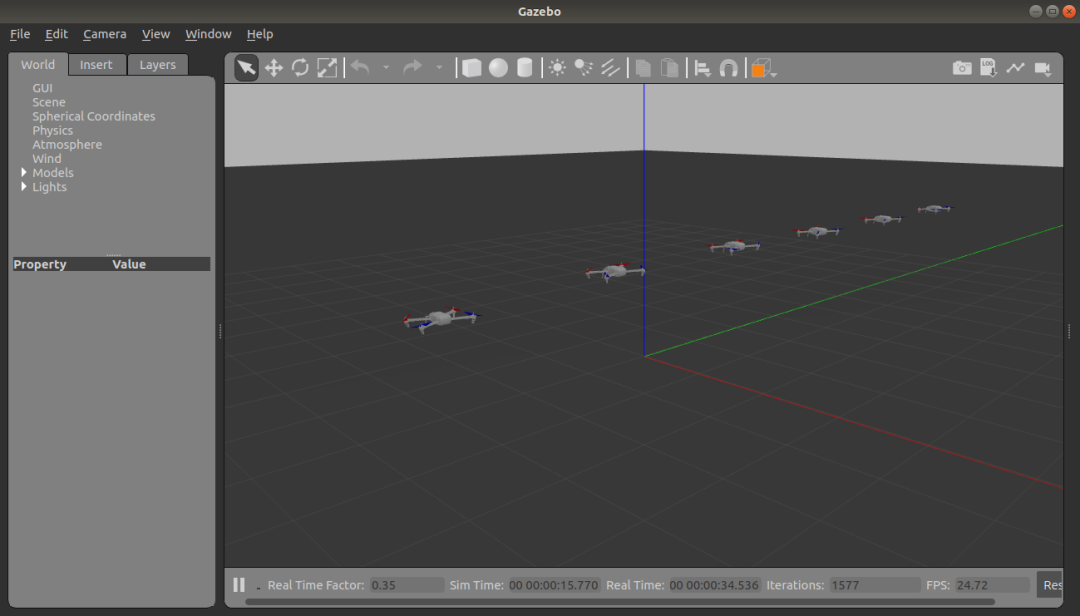那么我们怎么让这六架无人机按照我们想要的编队效果进行运动呢？这就需要我们写一个位置式的无人机控制器，通过读取Matlab仿真得到的无人机位置变化，分别对无人机进行控制，可以参考下面的python控制代码：rotors_formation_son.py#!/usr/bin/env python# -*- coding: utf-8 -*-#导入相关的库import rospyfrom geometry_msgs.msg import PoseStampedfrom geometry_msgs.msg import Twistfrom geometry_msgs.msg import Poseimport sys, select, termios, ttyfrom numpy import *import operatorfrom os import listdirimport time#建立结构体twist= Twist()pose=PoseStamped()#Create Publisher#初始化ros节点rospy.init_node('formation_publish')r=rospy.Rate(1)UAV=rospy.get_param('~UAV')seq=rospy.get_param('~seq')print(UAV+'command/pose')print(seq)num=seqpose_pub = rospy.Publisher(UAV+'command/pose',PoseStamped,queue_size=1)#初始化函数def __init__():        pose.pose.position.x=0        pose.pose.position.y=0        pose.pose.position.z=0#读取Matlab中的数据        def file2matrix(filename):    fr = open(filename)    numberOfLines = len(fr.readlines())         #get the number of lines in the file    returnMat = zeros((numberOfLines,3))        #prepare matrix to return    classLabelVector = []                       #prepare labels return       fr = open(filename)    index = 0    for line in fr.readlines():        line = line.strip()        listFromLine = line.split('\t')        classLabelVector.append(int(listFromLine))        returnMat[index,:] = listFromLine[1:4]        index += 1    return returnMat,numberOfLines #计算无人机位置    def odomCallback(msg):    global num    if msg.position.x>=pose.pose.position.x-0.1 and num        num += 6        time.sleep(0.01)        pose.pose.position.x=dataMat[num]        pose.pose.position.y=dataMat[num]*2        pose.pose.position.z=dataMat[num]*0.5        pose_pub.publish(pose)__init__();dataMat,numberOfLines = file2matrix("/home/zdz/source/data/pos_data.txt")#Create subscriberodom_sub = rospy.Subscriber('odometry_sensor1/pose',Pose, odomCallback,queue_size = 1)# spinrospy.spin()上述代码主要实现了读取Matlab中无人机编队的位置，并实时发布无人机位置的指令“command/pose”，最终通过分别给六架无人机发布位置式的指令，控制无人机的运动，达到编队飞行的效果(其中pos_data.txt是通过将Matlab中运行后得到的数据vehicle_pos转存为txt格式得到)最终运行起来的节点图如下所示：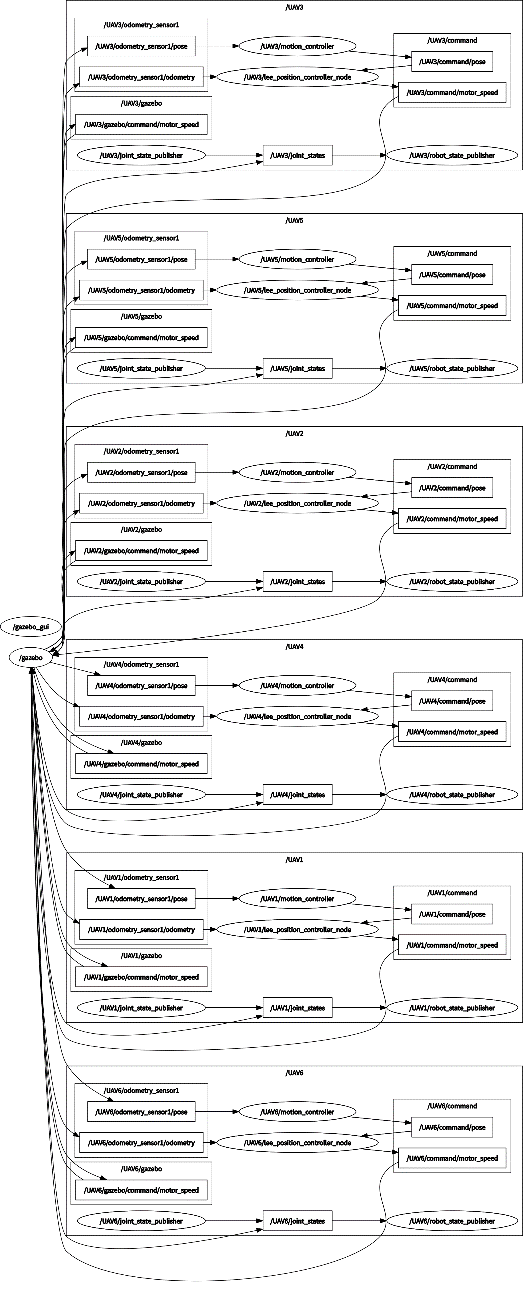多无人机编队仿真演示我们将编写的launch文件放入rotor_gazebo文件夹中，编译之后使用运行指令：roslaunch rotor_gazebo multi_uav_simulation.launch即可启动多无人机Gazebo仿真程序，多无人机编队飞行的效果可以参考下面录制的视频，在Gazebo仿真结果我们可以看到，六架无人机可以比较好的保持队形进行平移运动与旋转运动，大家有兴趣的话也可以自己动手尝试。期间有遇到任何问题可以在古月居论坛的无人机版块与笔者一起探讨。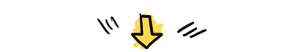《无人机仿真开发》课程提供的完整代码框架，在没有真实的无人机条件下，你将学到PX4无人机三维仿真开发，包含智能无人机开发仿真环境搭建、无人机自主飞行初步、无人机激光雷达避障自主飞行，方便大家做进一步的智能开发,涉及路径规划、自主决策等。在这个基础上实现强化学习，视觉追踪引导，激光/视觉SLAM等研发工作，本课程还提供一些例程DEMO提供完整的接口。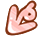点击“

展开全文gazebo安装
• 第4章-基于 PID 的无人机编队运动控制策略4.1 无人机编队条件假设4.2 无人机编队运动建模4.2.1 参考长机的选择4.2.2 参考坐标系的定义4.2.3 无人机质心运动方程4.2.4 相对运动模型4.3 模型分析4.4 基于 PID 的无人机...
• 也许是守望于浪漫主义的理想，也许是憧憬于无人机集群技术的未来潜力，阿木实验室的无人机编队技术开始实测啦！ 一、编队控制理论 对无人机协同控制的研究一般分为两个方向：编队构型的形成与构型维持。...
• 2020年，对于各行各业的人们来说都是艰难且不平凡的一年，无人机编队表演行业也不例外，受疫情影响，随着开业、庆典、上市、应援、推广造势等活动的取消，今年第一季度编队行业也按下了暂停键。 但随着国内疫情得到...
• 面向8-16岁中小学生，学生可自由选取音乐创作无人机编队舞蹈，运用图形化编程或代码实现超多空中编排队形；采用多机编队的震撼效果，加持多彩灯光的搭配，形成空中飞行动感屏幕，让编队表演更具...
• 第8章-危险状态下的无人机编队运动控制策略8.1 危险状态下的航迹规划问题8.2 基于层次分解策略的无人机编队避障8.2.1 编队避障行为控制层设计8.2.2 编队避障任务管理层设计8.2.3 编队避障航路控制器设计8.3 特殊情况...
• 第7章-基于多模型预测控制的无人机编队运动控制策略7.1 多无人机巡航编队控制系统设计思路7.2 基于多模型预测控制的无人机编队控制方法7.2.1 参考坐标系的选择7.2.2 无人机巡航编队飞行控制运动学模型7.2.3 无人机...
• px4下基于ros和gazebo的多无人机编队仿真，offboard模式 单机的offboard仿真见https://blog.csdn.net/weixin_43409270/article/details/114585397 多机仿真 1、修改launch文件 在/PX4-Autopilot/launch目录下，找到...linux
• 第6章-基于预测控制的无人机编队运动控制策略6.1 编队队形调节机制6.2 模型预测控制6.2.1 模型预测控制的发展6.2.2 模型预测控制的基本原理6.3 无人机编队队形控制6.3.1 编队相对运动分析6.3.2 编队队形控制器设计...
• 阿木实验室5架无人机编队齐飞 多机编队系统中，地面站和导航系统的作用不可或缺。导航系统决定飞机本省姿态和定位精度、决定队形保持的完美程度；普通的GPS无法满足队形精度，这里我们推荐了定位精度可以达到厘米...
• ## 说一说无人机编队的控制方法

万次阅读 多人点赞 2019-11-12 12:06:53
无人机、机器人编队或者是集群控制，说到底，虽然都是多智能体的控制，唯一有区别的在于空间或者平面，但随之带来的问题却衍生出不同的方向。在此稍微叙述下关于无人机的群体编队方面的。最早的研究是在60年代末，...
• 第5章-基于滑模控制的无人机编队运动控制策略5.1 编队数学模型5.2 控制策略与设计5.2.1 路径跟踪5.2.2 编队控制5.2.3 编队跟踪5.2.4 编队策略的扩展与改进5.3 仿真验证5.4 本章小结 滑模控制是一种具有抗干扰、稳定...
• 此处为一例程，使用两台电脑，每台电脑跑两架无人机无人机数量可格局需求自行调整 1、创建 SITL 环境 参考 https://blog.csdn.net/jzhd2015/article/details/108663961 2、配置多个无人机 单个电脑配置两个无人机...
• 中文摘要:无人机编队飞行可以大幅提高作战效率，因而受到了越来越多的关注。从近距离编队出发，采用长机-僚机编队结构，设计一种基于BP神经网络参数整定的PID控制器，并以双机菱形编队为基础，对其进行仿真验证。...
• 当每个无人机收到所有无人机的位置信息后，就可以根据整个编队的情况与自己现在的位置做出相应的调整。 调度的原则：始终保证中间组，若中间组损失，优先考虑调度两边不成组的，然后再考虑先调左边组，后调右边...
• % first row is lower and upper x bounds % second row is lower and upper y bounds %% Start updating the drones 开始更新无人机 close all; h = figure('Position', [10, 10, 800, 400]); % Do the initial ...
• 一、简介 人工势场法是局部路径规划的一种比较常用的方法。这种方法假设机器人在一种虚拟力场下运动。 如图所示，机器人在一个二维环境下运动，图中指出了机器人，障碍和目标之间的相对位置。 ......